# Right triangle - 9th grade (14y) - math problems

#### Number of problems found: 729

• In the 18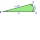In the right triangle ABC, The hypotenuse AB = 15 cm, and B = 25 degree. How long is BC to the nearest centimetre?
• Circle inscribed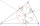There is a triangle ABC and a circle inscribed in this triangle with radius 15. The point T is the point of contact of the inscribed circle with the side BC. What is the area of the triangle ABC if | BT | = 25 a | TC | = 26?
• Bridge diagonally across the river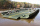The width of the river is 89 m. For terrain reasons, the bridge deviates from a common perpendicular to both banks by an angle of 12° 30 '. Calculate how many meters the bridge is longer than the river.
• TrapezoidsIn the isosceles trapezoid ABCD we know: AB||CD, |CD| = c = 8 cm, height h = 7 cm, |∠CAB| = 35°. Find the area of the trapezoid.
• Diagonals of rhombus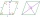Find a length of the diagonal AC of the rhombus ABCD if its perimeter P = 112 dm and the second diagonal BD has a length of 36 dm.
• One of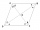One of the internal angles of the rhombus is 120° and the shorter diagonal is 3.4 meters long. Find the perimeter of the rhombus.
• Michael 2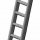Michael has a 35 foot ladder leaning against the side of his house. If the bottom of the ladder is 21 feet away from his house, how many feet above the ground does the ladder touch the house?
• Luiza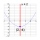Luiza delivers newspapers in her neighborhood. If you plot the points (-1, 1), (4, 1), (4, -2) and (-1, -2), you will create a representation of the route she takes, in miles. How many miles does her route cover?
• Centre of the hypotenuseFor the interior angles of the triangle ABC, alpha beta and gamma are in a ratio of 1: 2: 3. The longest side of the AB triangle is 30 cm long. Calculate the perimeter of the triangle CBS if S is the center of the side AB.
• Parallelogram diagonalsFind the area of a parallelogram if the diagonals u1 = 15 cm, u2 = 12 cm and the angle formed by them is 30 degrees.
• Center of gravity and medianIn the isosceles triangle ABC, the center of gravity T is 2 cm from the base AB. The median parallel to the AB side measures 4 cm. What is the area of the ABC triangle?
• MIT 1869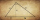You know the length of hypotenuse parts 9 and 16, at which the hypotenuse of a right triangle is divided by a perpendicular running from its opposite vertex. The task is to find the lengths of the sides of the triangle and the length of the line x. This a
• The gable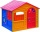The gable of the house has the shape of an isosceles triangle, which has a base length of 14 meters, and arms with a length of 8 meters. What is the tall gable of the house?
• Diamond and anglesFind the area of a diamond with a side of 5 cm if you know that the internal angles in the diamond are 60° and 120°.
• Isosceles trapezoidFind the height in an isosceles trapezoid if the area is 520 cm2 and the base a = 25 cm and c = 14 cm. Calculate the interior angles of the trapezoid.
• A rectangle 5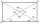A rectangle has sides of 10 cm and 14 cm. Calculate the angle between a diagonal and a long side.
• School modelThe beech school model of a regular quadrilateral pyramid has a base 20 cm long and 24 cm high. Calculate a) the surface of the pyramid in square decimeters, b) the mass of the pyramid in kilograms if the density of the beech is ρ = 0,8 g/cm ^ 3
• The rectangularThe rectangular trapezoid has bases 15 dm and 8 dm long and the length of the inclined arm is 12 dm. How long is the other arm of the trapezoid?
• The triangleThe triangle has sides 5 cm long, 5 cm, 8 cm long. What is the area of the triangle?
• Right-angled triangle baseFind the volume and surface area of a triangular prism with a right-angled triangle base if the length of the prism base legs are 7.2 cm and 4.7 cm and the height of a prism is 24 cm.

Do you have an exciting math question or word problem that you can't solve? Ask a question or post a math problem, and we can try to solve it.

We will send a solution to your e-mail address. Solved examples are also published here. Please enter the e-mail correctly and check whether you don't have a full mailbox.